# SAT Math Multiple Choice Question 4: Answer and Explanation

### Test Information

Question: 4

4.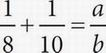In the equation above, if a and b are positive integers and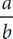is in its simplest reduced form, what is the value of a ?

• A. 2
• B. 9
• C. 18
• D. 40

B The lowest number that both 8 and 10 are factors of is 40. Convert the fractions to a denominator of 40: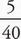+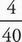=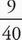. There is no factor that 9 and 40 have in common, so the fraction cannot be reduced. The number in place of a inis 9, so the answer is (B). Be careful! The value of b is in (D).